# Waste

How many percents are waste from a circular plate with a radius of 1 m from which we cut a square with the highest area?

p =  36.338 %

### Step-by-step explanation: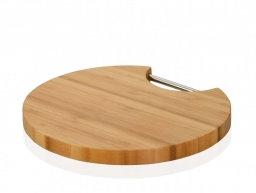Did you find an error or inaccuracy? Feel free to write us. Thank you!Tips to related online calculators
Pythagorean theorem is the base for the right triangle calculator.

#### You need to know the following knowledge to solve this word math problem:

We encourage you to watch this tutorial video on this math problem:

## Related math problems and questions:

• Cutting circlesFrom the square 1 m side we have to cut the circles with a radius of 10 cm. How many discs we cut and how many percent will be waste?
• Cutting square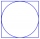From a square with a side of 30 cm, we cut the circle with the highest possible diameter. How many percents of the square content is this circle?
• Circular ringSquare with area 16 centimeters square are inscribed circle k1 and described circle k2. Calculate the area of circular ring, which circles k1, k2 form.
• Quarter circleWhat is the radius of a circle inscribed in the quarter circle with a radius of 100 cm?
• Square metal sheetWe cut out four squares of 300 mm side from a square sheet metal plate with a side of 0,7 m. Express the fraction and the percentage of waste from the square metal sheet.
• Percentage of waste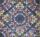In a square plate with side 75 cm we cut 4 same circles. Calculate the percentage of waste.
• Metal washersMetal washers with a diameter of 80 mm are cut from a strip of steel sheet with a width of 10 cm and a length of 2 m. Calculate the percentage of material waste if no material is lost when two adjacent circles meet.
• Roof 7The roof has the shape of a regular quadrangular pyramid with a base edge of 12 m and a height of 4 m. How many percent is folds and waste if in construction was consumed 181.4m2 of plate?
• Company logoThe company logo consists of a blue circle with a radius of 4 cm, which is an inscribed white square. What is the area of the blue part of the logo?
• Two circles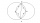Two circles with the same radius r = 1 are given. The center of the second circle lies on the circumference of the first. What is the area of a square inscribed in the intersection of given circles?
• BricklayerHow much do we pay for a bricklayer laying a pavement in a square room with a diagonal of 8 m, if 1 sqm with work will cost for CZK 420?
• FlakesWe describe a circle of the square, and we describe a semicircle above each side of the square. This created 4 flakes. Which is bigger: the area of the central square, or the area of four flakes?
• SquareCalculate the area of the square shape of the isosceles triangle with the arms 50m and the base 60m. How many tiles are used to pave the square if the area of one tile is 25 dm2?
• Sphere cutsAt what distance from the center intersects sphere with radius R = 56 plane, if the cut area and area of the main sphere circle is in ratio 1/2.
• Equilateral triangle vs circle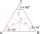Find the area of an equilateral triangle inscribed in a circle of radius r = 9 cm. What percentage of the circle area does it occupy?
• Ratio of sidesCalculate the area of a circle with the same circumference as the circumference of the rectangle inscribed with a circle with a radius of r 9 cm so that its sides are in ratio 2 to 7.
• GardenThe area of a square garden is 2/9 of triangle garden with sides 160 m, 100 m, and 100 m. How many meters of fencing need to fence a square garden?